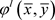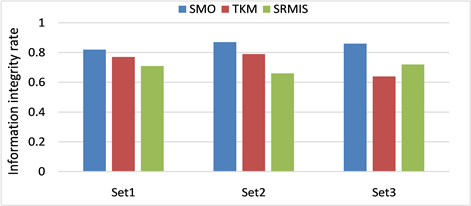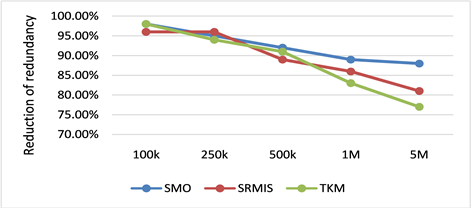﻿ Web数据转换模式映射优化方法

# Web数据转换模式映射优化方法Web Data Exchange Schema Mapping Optimization Method

Abstract: Web data exchange is one of the important researches on the integration of Web heterogeneous data sources. It is usually divided into two aspects: instance layer and schema layer. The research in this paper is mainly focused on the mode layer. Because a given source-to-target mode mapping usually makes the data exchange results contain a lot of redundancy, in order to generate data without redundancy as a data exchange kernel solution, this paper designs a homomorphic rela-tionship Schema mapping design and optimization methods. This method first introduces the ho-momorphic relationship between the schema mappings as the basis of the schema mapping re-writing method. By decomposing the schema mappings, defining the degree of data redundancy generated by different rules, and determining the rules that need to be rewritten. Finally, the given schema mapping is rewritten into a kernel schema mapping that can directly generate a kernel so-lution, and it is converted into an executable SQL statement to calculate the kernel solution. This paper uses data from China Land Market Network to test the performance of the proposed method.

1. 引言Table 1. Source database summary dataTable 2. Target database summary data

m1. $\forall Pname,Paddr:B\left(Pname,Paddr\right)\to \exists I:T1\left(Pname,I\right)\wedge T2\left(I,Paddr\right)$

m2. $\forall Pname,Pnumber:C\left(Pname,Pnumber\right)\to T1\left(Pname,Pnumber\right)$

m3. $\forall Pnumber,Paddr:D\left(Pnumber,Paddr\right)\to T2\left(Pnumber,Paddr\right)$

m4. $\forall Pname:A\left(Pname\right)\to \exists N:T1\left(Pname,N\right)$

1) 本文将给定映射规则目标模式上的查询定义为扩展，表示满足映射规则的所有查询公式的集合，它们可以作为一阶查询来捕获目标实例。将矩阵之间的同态关系概念应用到扩展中，通过扩展之间的同态关系，查找到目标实例中满足核解特征的目标实例，扩展间的同态关系称为公式同态。

2) 每当为给定映射规则确定了一个比基扩展更合适的扩展时，可以根据以下策略执行映射规则来防止在目标中生成冗余元组。首先通过更合适的扩展查找目标实例，然后使用基扩展只生成那些实际向目标添加一些新内容的目标实例。通过这种方式，我们可以从扩展的角度对核解的特征进行计算，这是本文重写算法的基础。

3) 为了将上述研究转化为实际的重写方法，通过将扩展重写为源模式上的查询公式，扩展的源重写会在源数据库上声明一个条件，以生成目标中与扩展 $\epsilon$ 相关的目标实例。在我们的例子中，扩展的源重写如下：

$sourceRew\left(T1\left(Pname,Pnumber\right)\right)=IBLBook\left(t,id\right)$

$sourceRewT1\left(Pname,Pnumber\right)\wedge T2\left(Pnumber,Paddr\right)=C\left(Pname,Pnumber\right),D\left(Pnumber,Paddr\right)$

4) 通过本文的示例研究发现，并不是所有规则都会导致目标中生成冗余实例。在对原始规则进行重写时，应去除那些不含存在量词并且源到目标对应关系明确的规则，为此我们给出初始规则选择方法，只选择那些会生成冗余元组的规则进行重写。分析给定的映射规则集，为每条规则分配一个冗余度，用来确定生成目标实例冗余程度，以便识别其中的哪条规则可能在目标中生成冗余元组。

5) 最终根据本文方法可以生成以下规则，它们比普通的映射规则更具表现力，允许在前提中添加否定，可以用来表示原始场景的核心模式映射，通过在源实例上执行这些规则能够生成核解，其中r1、r4为重写后的规则，r2、r3为生成目标数据精确度较高的规则，未进行重写：

r1. $\forall {x}_{1},{x}_{2}:B\left({x}_{1},{x}_{2}\right)\wedge ¬\left(\exists {x}_{4},{x}_{5}:C\left({x}_{1},{x}_{4}\right)\wedge D\left({x}_{5},{x}_{2}\right)\wedge {x}_{4}={x}_{5}\right)\to T1\left({x}_{1},{f}_{{x}_{1}}\right)\wedge T2\left({f}_{{x}_{2}},{x}_{2}\right)$

r2. $C\left({x}_{3},{x}_{4}\right)\to T1\left({x}_{3},{x}_{4}\right)$

r3. $D\left({x}_{5},{x}_{6}\right)\to T2\left({x}_{5},{x}_{6}\right)$

r4. $\forall {x}_{7},A\left({x}_{7}\right)\wedge ¬\left(\exists {x}_{2}:B\left({x}_{7},{x}_{2}\right)\right)\wedge ¬\left(\exists {x}_{4}:C\left({x}_{7},{x}_{4}\right)\right)\to T1\left({x}_{7},{f}_{{x}_{7}}\right)$

2. 相关概念

i) ${h}^{f}$${\phi }^{l}\left(\stackrel{¯}{x},\stackrel{¯}{y}\right)$ 中的全称量词映射为 ${{\phi }^{\prime }}^{l}\left({\stackrel{¯}{x}}^{\prime },{\stackrel{¯}{y}}^{\prime }\right)$ 中的全称量词；

ii) 对于每个原子 ${R}^{l}\left({A}_{1}:{v}_{1},\cdots ,{A}_{k}:{v}_{k}\right)\in {\phi }^{l}\left(\stackrel{¯}{x},\stackrel{¯}{y}\right)$，在集合 ${{\phi }^{\prime }}^{l}\left({\stackrel{¯}{x}}^{\prime },{\stackrel{¯}{y}}^{\prime }\right)$ 上存在 $R\left({h}^{f}\left({R}^{l}\left({A}_{1}:{v}_{1},\cdots ,{A}_{k}:{v}_{k}\right)\in {{\phi }^{\prime }}^{l}\left({\stackrel{¯}{x}}^{\prime },{\stackrel{¯}{y}}^{\prime }\right)\right)\right)$ 与之对应；

iii) 对于存在变量 $y\in \stackrel{¯}{y}$，变量 ${R}_{n}^{l}.{A}_{m}:y$${R}_{i}^{l}.{A}_{j}:y$ 成对出现，在这种情况下， ${h}^{f}\left({R}_{n}^{l}.{A}_{m}:y\right)$${h}^{f}\left({R}_{i}^{l}.{A}_{j}:y\right)$ 都是常量，或者是在 ${y}^{\prime }\in {\stackrel{¯}{y}}^{\prime }$ 中相同的存在变量。

${\phi }^{l}={R}^{1}\left({x}_{1},{x}_{2},{Y}_{1}\right)\wedge {T}^{2}\left({x}_{3},{x}_{1},{Y}_{1}\right)$${{\phi }^{\prime }}^{l}={R}^{3}\left({{x}^{\prime }}_{4},{{x}^{\prime }}_{5},{{x}^{\prime }}_{6}\right)\wedge {T}^{4}\left({{x}^{\prime }}_{9},{{x}^{\prime }}_{7},{{x}^{\prime }}_{8}\right)$

${h}^{f}\left({R}^{1}.A:{x}_{1}\right)\to {R}^{3}.A:{{x}^{\prime }}_{4}$${h}^{f}\left({R}^{1}.B:{x}_{2}\right)\to {R}^{3}.B:{{x}^{\prime }}_{5}$

${h}^{f}\left({R}^{1}.C:{Y}_{1}\right)\to {R}^{3}.C:{{x}^{\prime }}_{6}$${h}^{f}\left({T}^{2}.A:{x}_{3}\right)\to {T}^{4}.A:{{x}^{\prime }}_{9}$

${h}^{f}\left({T}^{2}.B:{x}_{1}\right)\to {T}^{4}.B:{{x}^{\prime }}_{7}$${h}^{f}\left({T}^{2}.C:{Y}_{1}\right)\to {T}^{4}.C:{{x}^{\prime }}_{8}$

i) 如果 ${h}^{f}$ 是满射的，则公式同态 ${h}^{f}$ 是更紧凑的。可以是 $|{{\phi }^{\prime }}^{l}\left({\stackrel{¯}{x}}^{\prime },{\stackrel{¯}{y}}^{\prime }\right)|<|{\phi }^{l}\left(\stackrel{¯}{x},\stackrel{¯}{y}\right)|$$|{\stackrel{¯}{y}}^{\prime }|<|\stackrel{¯}{y}|$ 的情况。即要么 ${{\phi }^{\prime }}^{l}\left({\stackrel{¯}{x}}^{\prime },{\stackrel{¯}{y}}^{\prime }\right)$${\phi }^{l}\left(\stackrel{¯}{x},\stackrel{¯}{y}\right)$ 小，要么 ${{\phi }^{\prime }}^{l}\left({\stackrel{¯}{x}}^{\prime },{\stackrel{¯}{y}}^{\prime }\right)$ 包括较少的存在变量；

ii) 如果 ${h}^{f}$ 是单射的而不是满射的，则 ${h}^{f}$ 被认为是更适当的，即在 ${{\phi }^{\prime }}^{l}\left({\stackrel{¯}{x}}^{\prime },{\stackrel{¯}{y}}^{\prime }\right)$ 中至少有一个原子不是的原子的图像。

$INTERSEC{T}_{{h}^{f}}$ 表示 ${\phi }^{l}\left(\stackrel{¯}{x},\stackrel{¯}{y}\right)$${{\phi }^{\prime }}^{l}\left({\stackrel{¯}{x}}^{\prime },{\stackrel{¯}{y}}^{\prime }\right)$ 之间全称量词的一组等价集(等式集合)，这组等式必须成立，才能实现这两个公式实例之间的同态。

$INTERSEC{T}_{{h}^{f}}\left(\stackrel{¯}{x},{\stackrel{¯}{x}}^{\prime }\right)=\left\{{x}_{i}={{x}^{\prime }}_{j}|{h}^{f}\left(R.A:{x}_{i}\right)=R.A:{{x}^{\prime }}_{j},{x}_{i}\in \stackrel{¯}{x},{{x}^{\prime }}_{j}\in \stackrel{¯}{y}\right\}$

$JOIN{S}_{{h}^{f}}$ 表示 ${{\phi }^{\prime }}^{l}\left({\stackrel{¯}{x}}^{\prime },{\stackrel{¯}{y}}^{\prime }\right)$ 的全称量词之间的等价集，其具体的值是 ${\phi }^{l}\left(\stackrel{¯}{x},\stackrel{¯}{y}\right)$ 中相同存在量词的值的图像。

$JOIN{S}_{{h}^{f}}\left(\stackrel{¯}{x}\right)=\left\{{{x}^{\prime }}_{h}={{x}^{\prime }}_{l}|{{x}^{\prime }}_{h}={h}_{{R}_{i}.{A}_{j}}^{f}\left({y}_{k}\right),{{x}^{\prime }}_{l}={h}_{{R}_{n}.{A}_{m}}^{f}\left({y}_{k}\right),{y}_{k}\in \stackrel{¯}{y}\right\}$

$EQUA{L}_{{h}^{f}}\left(\stackrel{¯}{x},{\stackrel{¯}{x}}^{\prime }\right)=INTERSEC{T}_{{h}^{f}}\left(\stackrel{¯}{x},{\stackrel{¯}{x}}^{\prime }\right)\cup JOIN{S}_{{h}^{f}}\left({\stackrel{¯}{x}}^{\prime }\right)$

3. 模式映射重写

3.1. 扩展

$J|={a}_{1}\left({\chi }^{l}\left({\stackrel{¯}{x}}_{1},{\stackrel{¯}{y}}_{1}\right)\right)$

${a}_{1},{a}_{2}$$EQUA{L}_{{h}^{f}}\left({a}_{1}\left({\stackrel{¯}{x}}_{1}\right),{a}_{2}\left({\stackrel{¯}{x}}_{2}\right)\right)$ 的形式。

3.1.1. 扩展与核解

3.1.2. 扩展的分类

$\epsilon ={\chi }^{l}\left({\stackrel{¯}{x}}_{1},{\stackrel{¯}{y}}_{1}\right)\wedge \exists {\stackrel{¯}{x}}_{2},{\stackrel{¯}{y}}_{2}:\left({\psi }^{l}\left({\stackrel{¯}{x}}_{2},{\stackrel{¯}{y}}_{2}\right)\wedge EQUA{L}_{{h}_{c}^{f}}\left({\stackrel{¯}{x}}_{1},{\stackrel{¯}{x}}_{2}\right)\right)$

${\epsilon }^{\prime }={{\chi }^{\prime }}^{l}\left({{\stackrel{¯}{x}}^{\prime }}_{1},{{\stackrel{¯}{y}}^{\prime }}_{1}\right)\wedge \exists {{\stackrel{¯}{x}}^{\prime }}_{2},{{\stackrel{¯}{y}}^{\prime }}_{2}:\left({{\psi }^{\prime }}^{l}\left({{\stackrel{¯}{x}}^{\prime }}_{2},{{\stackrel{¯}{y}}^{\prime }}_{2}\right)\wedge EQUA{L}_{{h}_{c\text{'}}^{f}}\left({{\stackrel{¯}{x}}^{\prime }}_{1},{{\stackrel{¯}{x}}^{\prime }}_{2}\right)\right)$

3.1.3. 扩展的选择

$\epsilon ={\chi }^{l}\left({\stackrel{¯}{x}}_{1},{\stackrel{¯}{y}}_{1}\right)\wedge \exists {\stackrel{¯}{x}}_{2},{\stackrel{¯}{y}}_{2}:\left({\psi }^{l}\left({\stackrel{¯}{x}}_{2},{\stackrel{¯}{y}}_{2}\right)\wedge EQUA{L}_{{h}_{c}^{f}}\left({\stackrel{¯}{x}}_{1},{\stackrel{¯}{x}}_{2}\right)\right)$

$mostComp\left(\epsilon \right)$ 通过如下方式获得：

1) 初始化 $mostComp\left(\epsilon \right)=\epsilon$

2) 对于 $expansions\left(M\right)$ 中的任意 ${\epsilon }^{\prime }={{\chi }^{\prime }}^{l}\left({{\stackrel{¯}{x}}^{\prime }}_{1},{{\stackrel{¯}{y}}^{\prime }}_{1}\right)\wedge \exists {{\stackrel{¯}{x}}^{\prime }}_{2},{{\stackrel{¯}{y}}^{\prime }}_{2}:\left({{\psi }^{\prime }}^{l}\left({{\stackrel{¯}{x}}^{\prime }}_{2},{{\stackrel{¯}{y}}^{\prime }}_{2}\right)\wedge EQUA{L}_{{h}_{c\text{'}}^{f}}\left({{\stackrel{¯}{x}}^{\prime }}_{1},{{\stackrel{¯}{x}}^{\prime }}_{2}\right)\right)$ 和任意形如 $\epsilon {\prec }_{{h}^{f}}{\epsilon }^{\prime }$ 的公式同态 ${h}^{f}$。即 ${\epsilon }^{\prime }$ 根据 ${h}^{f}$ 变得比 $\epsilon$ 更紧凑，向 $mostComp\left(\epsilon \right)$ 添加公式 $\wedge ¬\exists {{\stackrel{¯}{x}}^{\prime }}_{1},{{\stackrel{¯}{y}}^{\prime }}_{1}:\left({\epsilon }^{\prime }\wedge EQUA{L}_{{h}_{c}^{f}}\left({\stackrel{¯}{x}}_{1},{{\stackrel{¯}{x}}^{\prime }}_{1}\right)\right)$

$\epsilon ={\chi }^{l}\left({\stackrel{¯}{x}}_{1},{\stackrel{¯}{y}}_{1}\right)\wedge \exists {\stackrel{¯}{x}}_{2},{\stackrel{¯}{y}}_{2}:\left({\psi }^{l}\left({\stackrel{¯}{x}}_{2},{\stackrel{¯}{y}}_{2}\right)\wedge EQUA{L}_{{h}_{c}^{f}}\left({\stackrel{¯}{x}}_{1},{\stackrel{¯}{x}}_{2}\right)\right)$

$mostInf\left(\epsilon \right)$ 的具体操作如下：

1) 初始化 $mostInf\left(\epsilon \right)=mostComp\left(\epsilon \right)$

2) 对于 $expansions\left(M\right)$ 中的任意扩展

${\epsilon }^{\prime }={{\chi }^{\prime }}^{l}\left({{\stackrel{¯}{x}}^{\prime }}_{1},{{\stackrel{¯}{y}}^{\prime }}_{1}\right)\wedge \exists {{\stackrel{¯}{x}}^{\prime }}_{2},{{\stackrel{¯}{y}}^{\prime }}_{2}:\left({{\psi }^{\prime }}^{l}\left({{\stackrel{¯}{x}}^{\prime }}_{2},{{\stackrel{¯}{y}}^{\prime }}_{2}\right)\wedge EQUA{L}_{{h}_{c\text{'}}^{f}}\left({{\stackrel{¯}{x}}^{\prime }}_{1},{{\stackrel{¯}{x}}^{\prime }}_{2}\right)\right)$ 和任意形如 $\epsilon {<}_{{h}^{f}}{\epsilon }^{\prime }$ 的公式同态 ${h}^{f}$，即 ${\epsilon }^{\prime }$${h}^{f}$ 变得比 $\epsilon$ 更具信息性，向 $mostInf\left(\epsilon \right)$ 添加公式

$\wedge ¬\exists {{\stackrel{¯}{x}}^{\prime }}_{1},{{\stackrel{¯}{y}}^{\prime }}_{1}:\left(mostComp\left({\epsilon }^{\prime }\right)\wedge EQUA{L}_{{h}_{c}^{f}}\left({\stackrel{¯}{x}}_{1},{{\stackrel{¯}{x}}^{\prime }}_{1}\right)\right)$

a) 首先通过添加所有的扩展 ${\epsilon }_{i}$ 否定，将 $\epsilon$ 重写成一个新形式： $mostComp\left(\epsilon \right)$${\epsilon }_{i}$$\epsilon$ 更紧凑；本文期望用这些新的公式选择在与 $\epsilon$ 相关的目标实例中更紧凑的；

b) 然后通过添加 $mostComp\left({\epsilon }_{j}\right)$ 的否定，进一步将 $mostComp\left(\epsilon \right)$ 重写为一个新的公式 $mostInf\left(\epsilon \right)$，扩展 ${\epsilon }_{j}$$\epsilon$ 更具信息性。

3.2. 规则选择

3.2.1. 规则冗余程度

$J\left(A,B\right)=\frac{|A\cap B|}{|A\cup B|}=\frac{|A\cap B|}{|A|+|B|-|A\cap B|}$

3.2.2. 规则分解

1) 1:1映射关系

1:1映射关系是指web数据源模式与目标数据模式中的表结构是一致的，即标准数据库中的某些表在源数据库中有唯一的一个数据与之对应。对于映射关系为1:1的映射规则，若其目标模式中不含存在量词，则不要进行重写，直接作为核模式映射输出。若其目标模式中包含存在量词，这些规则生成的数据中必定包含较多的变量，说明这些规则是冗余的，直接进入待重写的规则库。

2) 1:N映射表关系

1:N映射关系是指web数据源模式与目标数据模式的表结构并非是一致的，即源数据库中原始数据的一个表映射到目标数据库中的多个表。首先将其分解为1:1类型的映射，然后根据其包含存在量词的数量来确定其冗余程度的大小，判断是否需要对其进行重写。

3) N:M映射表关系

N:M映射关系是指web数据源模式与目标数据模式的表结构并非是一致的，即源数据库中原始数据的多个表映射到目标数据库中的多个表。当规则中的映射关系为M:N时，可以首先将其转换成M个1:N的映射关系；然后再将1:N的映射处理为N个1:1之间的映射；最后对M * N个1:1的映射关系进行判断处理即可。在其中若不包含存在量词，则作为核心模式映射输出；若其中包含存在量词，则其冗余度较大，需要按照本文方法进行重写，转换成核心模式映射来计算核解。

3.3. 重写方法

3.3.1. 源重写

$premise\left({\chi }^{l}\left({\stackrel{¯}{x}}_{1},{\stackrel{¯}{y}}_{1}\right)\right)=\wedge \left\{premise\left({R}_{i}^{l}\left({\stackrel{¯}{x}}_{i},{\stackrel{¯}{y}}_{i}\right)\right)|{R}_{i}^{l}\left({\stackrel{¯}{x}}_{i},{\stackrel{¯}{y}}_{i}\right)\in {\chi }^{l}\left({\stackrel{¯}{x}}_{1},{\stackrel{¯}{y}}_{1}\right)\right\}$

$SourceRew\left(\epsilon \right)=premise\left({\chi }^{l}\left({\stackrel{¯}{x}}_{1},{\stackrel{¯}{y}}_{1}\right)\right)\wedge \exists {\stackrel{¯}{x}}_{2}:\left(\varphi \left({\stackrel{¯}{x}}_{2}\right)\wedge EQUA{L}_{{h}_{c}^{f}}\left({\stackrel{¯}{x}}_{1},{\stackrel{¯}{x}}_{2}\right)\right)$

3.3.2. 源重写的否定

i) 首先初始化

$SourceRew\left(mostComp\left(\epsilon \right)\right)=SourceRew\left(\epsilon \right)$

ii) 然后对于扩展集 $expansions\left(M\right)$ 中的任何扩展 ${\epsilon }^{\prime }$，若 ${\epsilon }^{\prime }$ 是比 $\epsilon$ 更紧凑的，称 ${h}_{c}^{f}$ 为从 ${\chi }^{l}\left({\stackrel{¯}{x}}_{1},{\stackrel{¯}{y}}_{1}\right)$${{\chi }^{\prime }}^{l}\left({{\stackrel{¯}{x}}^{\prime }}_{1},{{\stackrel{¯}{y}}^{\prime }}_{1}\right)$ 的更紧凑的同态；向 $SourceRew\left(mostComp\left(\epsilon \right)\right)$ 添加一个公式：

$\wedge ¬\exists {\stackrel{¯}{x}}^{\prime }:\left(SourceRew\left({\epsilon }^{\prime }\right)\wedge EQUA{L}_{{h}_{c}^{f}}\left({\stackrel{¯}{x}}_{1},{{\stackrel{¯}{x}}^{\prime }}_{1}\right)\right)$

i) 初始化

$SourceRew\left(mostInf\left(\epsilon \right)\right)=SourceRew\left(mostComp\left(\epsilon \right)\right)$

ii) 然后对于扩展集 $expansions\left(M\right)$ 中的任何扩展 ${\epsilon }^{\prime }$，若 ${\epsilon }^{\prime }$ 是比 $\epsilon$ 更具信息性的，称 ${h}_{c}^{f}$ 为从 ${\chi }^{l}\left({\stackrel{¯}{x}}_{1},{\stackrel{¯}{y}}_{1}\right)$${{\chi }^{\prime }}^{l}\left({{\stackrel{¯}{x}}^{\prime }}_{1},{{\stackrel{¯}{y}}^{\prime }}_{1}\right)$ 的更恰当的同态；向 $SourceRew\left(mostInf\left(\epsilon \right)\right)$ 添加一个公式：

$\wedge ¬\exists {{\stackrel{¯}{x}}^{\prime }}_{1}:\left(SourceRew\left(mostComp\left({\epsilon }^{\prime }\right)\right)\wedge EQUA{L}_{{h}_{c}^{f}}\left({\stackrel{¯}{x}}_{1},{{\stackrel{¯}{x}}^{\prime }}_{1}\right)\right)$

3.3.3. 重写算法

$\forall {\stackrel{¯}{x}}_{1}:SourceRew\left(mostInf\left(\epsilon \right)\right)\left({\stackrel{¯}{x}}_{1}\right)\to {\chi }_{i}\left({\stackrel{¯}{x}}_{1},{\stackrel{¯}{y}}_{1}\right)$

${\text{Σ}}_{M}^{e}=\left\{expansionRule\left(\epsilon \right)|\epsilon \in expansions\left(M\right)\right\}$

i) 首先初始化 $finalRew\left(r\right)=r$

ii) 对于 ${\text{Σ}}_{M}^{e}$ 中的任意规则 ${r}^{\prime }.{\varphi }^{\prime }\left({x}^{\prime }\right)\to {\psi }^{\prime }\left({x}^{\prime }\right)$，根据同态 ${h}_{i}^{f}$ 说明 ${\psi }^{\prime }\left({x}^{\prime }\right)$ 是比 $\psi \left(x\right)$ 更具信息性的，向 $finalRew\left(r\right)$ 增加一个前提公式 $\wedge ¬\exists \left({\stackrel{¯}{x}}^{\prime }\right):\left({\varphi }^{\prime }\left({x}^{\prime }\right)\wedge EQUA{L}_{{h}_{i}^{f}}\left(\stackrel{¯}{x},\stackrel{¯}{{x}^{\prime }}\right)\right)$

${\text{Σ}}_{M}^{c}=\left\{finalRew\left(r\right)|r\in {\text{Σ}}_{M}^{e}\right\}$

4. 实验结果

4.1. 数据集Table 3. Real estate information data sheet attribute descriptionTable 4. Property description of the Weibo data table

4.2. 实验设置

$\text{Precision}=\frac{满足核解特征的实例数}{目标数据库中存储实例总数}×100\text{%}$

$\text{Reduction}\text{\hspace{0.17em}}\text{of}\text{\hspace{0.17em}}\text{repetition}=\frac{最终生成数据冗余度}{初始冗余程度}×100\text{%}$

4.3. 实验结果Figure 1. Information integrity comparisonFigure 2. Reduction of redundancy

5. 结论

 Fagin, R., Kolaitis, P.G., et al. (2003) Data Exchange: Semantics and Query Answering. In: Database Theory—ICDT 2003, Springer, Berlin, Heidelber, 207-224. https://doi.org/10.1007/3-540-36285-1_14

 Fagin, R., Kolaitis, P.G. and Popa, L. (2005) Data Exchange: Getting to the Core. ACM Transactions on Database Systems, 30, 174-210. https://doi.org/10.1145/1061318.1061323

 Pichler, R. and Savenkov, V. (2010) Towards Practical Feasibility of Core Computation in Data Exchange. Theoretical Computer Science, 411, 935-957. https://doi.org/10.1016/j.tcs.2009.09.035

 Kimmig, A., Memory, A., Miller, R.J. and Getoor, L. (2017) A Collective, Probabilistic Approach to Schema Mapping. 2017 IEEE 33rd International Conference on Data Engineering (ICDE), San Diego, CA, 19-22 April 2017, 921-932. https://doi.org/10.1109/ICDE.2017.140

 Gottlob, G. and Nash, A. (2006) Data Exchange: Computing Cores in Polynomial Time. ACM Sigmod-Sigact-Sigart Symposium on Principles of Database Systems, June 2006, 40-49. https://doi.org/10.1145/1142351.1142358

 Yousfi, A., Elyazidi, M.H. and Zellou, A. (2018) Assessing the Performance of a New Semantic Similarity Measure Designed for Schema Matching for Mediation Systems. In: International Conference on Computational Collective Intelligence, Springer, Cham, 64-74. https://doi.org/10.1007/978-3-319-98443-8_7

 Kettouch, M., Luca, C. and Hobbs, M. (2017) Schema Matching for Semi-structured and Linked Data. 2017 IEEE 11th International Conference on Semantic Computing (ICSC), San Diego, CA, 30 January-1 February 2017, 270-271. https://doi.org/10.1109/ICSC.2017.104

 Sekhavat, Y.A. and Parsons, J. (2017) SEDEX: Scalable Entity Preserving Data Exchange. 2017 IEEE 33rd International Conference on Data Engineering (ICDE), San Diego, CA, 19-22 April 2017, 65-66. https://doi.org/10.1109/ICDE.2017.39

 Alexe, B., ten Cate, B., Kolaitis, P.G. and Tan, W. (2011) EIRENE: Interactive Design and Refinement of Schema Mappings via Data Examples. Proceedings of the VLDB Endowment, 4, 1414-1417. https://doi.org/10.1145/2043652.2043656

 Alexe, B., Hernndez, M., Popa, L. and Tan, W.C. (2012) MapMerge: Correlating Independent Schema Mappings. The VLDB Journal, 21, 191-211. https://doi.org/10.1007/s00778-012-0264-z

 解筱, 张克, 任伯群, 等. ETL技术在商业银行数据整合中的研究与应用[J]. 信息技术与信息化, 2019(7): 45-47.

 丁强龙, 王津, 张学杰. 基于子模式的关系数据到图数据ETL方法研究[J]. 计算机工程与应用, 2017, 53(12): 76-84.

 李磊. ETL任务集群调度方法[J]. 计算机技术与发展, 2018, 28(11): 41-44.

 Baker, C.A. (1995) Extended Skolem Sequences. Journal of Combinatorial Designs, 3, 363-379. https://doi.org/10.1002/jcd.3180030507

 Ravichandra, S. and Somayajulu, D.V.L.N. (2015) Core Schema Mappings: Computing Core Solution with Target Dependencies in Data Exchange.

 吕劲松, 王忠. 金融审计中的数据分析[J]. 审计研究, 2014(5): 28-33.

 Fan, H., Deng, K. and Liu, J. (2016) An Approach of XML Schema Matching Using Top-K Mapping. 2016 3rd International Conference on Information Science & Control Engineering (ICISCE), Beijing, 8-10 July 2016, 174-178. https://doi.org/10.1109/ICISCE.2016.47

 Hsu, I.C., Yang, L.J., Huang, D.C., et al. (2014) Integrating Semantic Web Technologies with XML Schema Using Role-Mapping Annotations. The Electronic Library, 32, 147-169. https://doi.org/10.1108/EL-07-2012-0096

Top